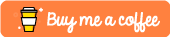# Perform your favorite data analysis in Python

## Chapter 0: Introduction to the Course

Is this course worth your time? Let's find out!

## Chapter 1: Python Basics

In this chapter, we are going to learn how to define variables in Python and what are the main data types.

## Chapter 2: Programming Fundamentals

In this chapter, we are going to look at programming concepts, such as working with loops, writing custom functions, and dealing with errors.

## Chapter 3: Packages for Data Manipulation

We will introduce two main packages for working with data. We are going to see how we can use NumPy to perform linear algebra calculations and how to perform data manipulations on tabular data using Pandas.

## Chapter 4: Data Visualization

Making plots from the raw data is a powerful and somewhat underestimated tool that could give you a better understanding of what is going on in your data and some interesting insights. In this chapter we will look at the main plot types and how to create them in Python using Matplotlib and Seaborn packages.

## Chapter 5: I/O

Now that we know how to work with data it's time to learn how to actually import the data from external sources and files into Python. We will see how to load files of different formats, such as txt, csv, mat, and how to create API requests.

## Chapter 6: Statistics in Python

We will look at how to perform statistical analysis such as ANOVA or Chi-squared test using mainly scipy.stats module and pingouin package.

## Chapter 7: More Examples

Miscellaneous examples of other awesome stuff you could do with Python to deal with "real-world" problems.